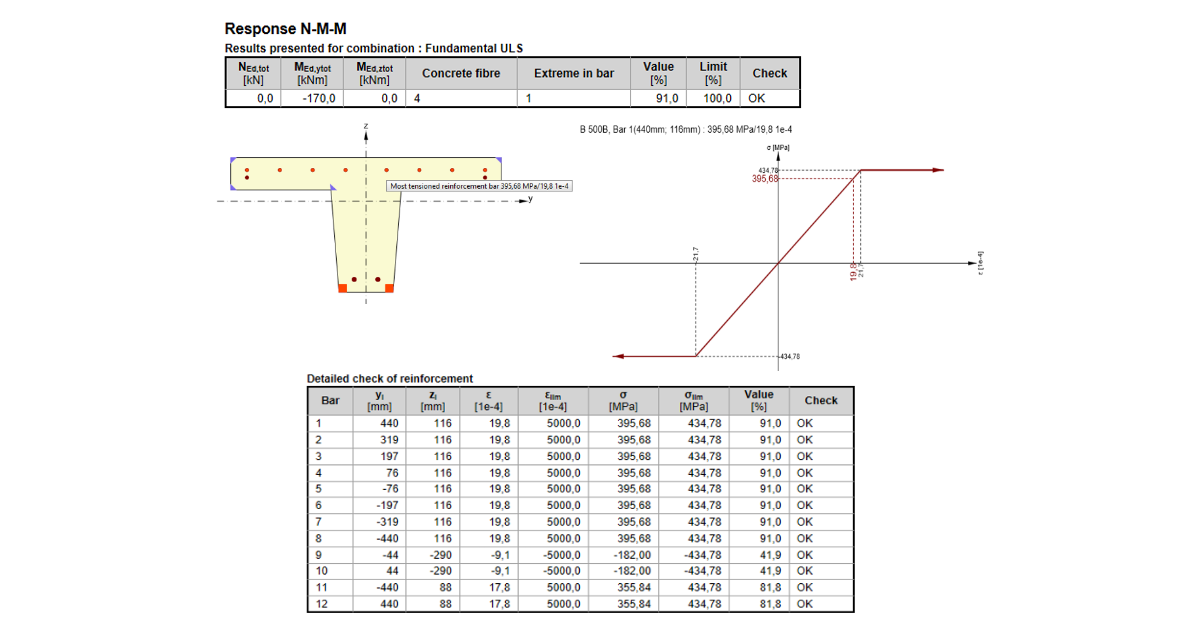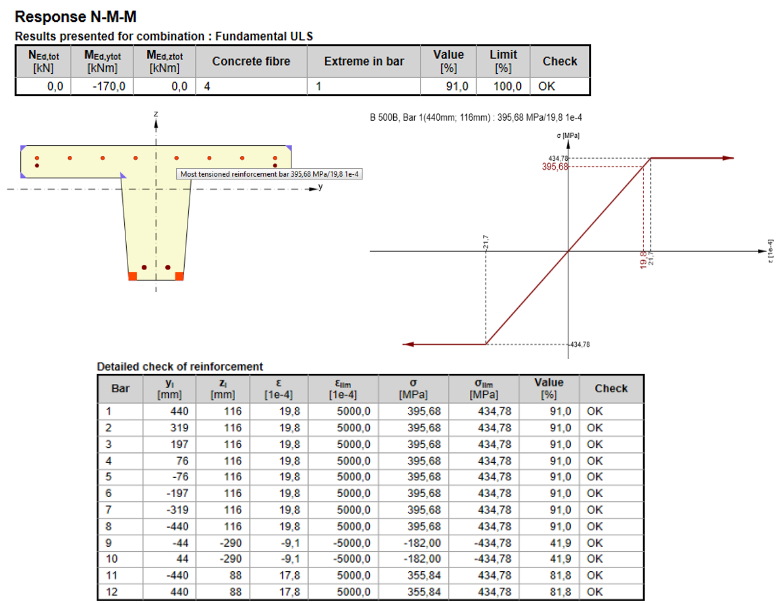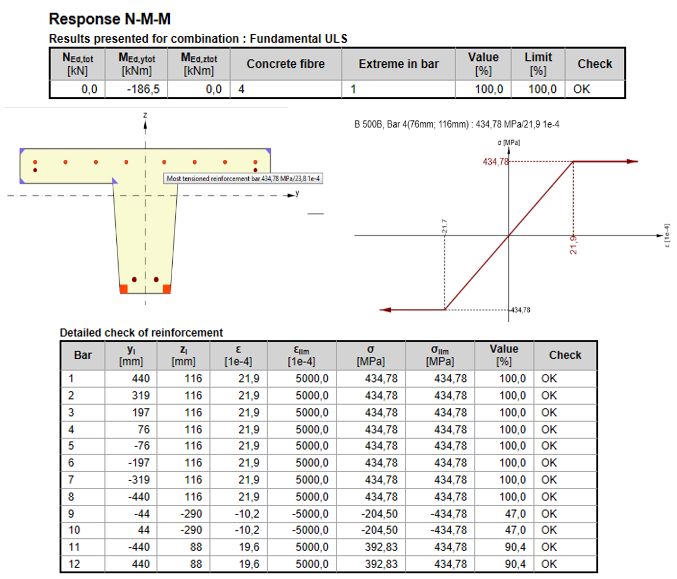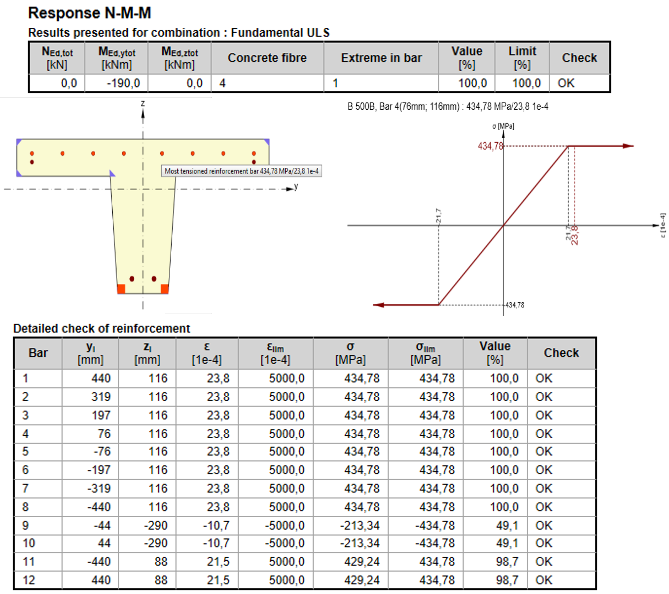### Choose language# Check of the cross-section by Response N-M-M?

$$If we find the equilibrium of a cross-section, we can determine the plane of strain. We can calculate the strain anywhere along the depth of the cross-section based on this plane. Using the stress-strain diagrams for each material we receive the stresses, respectively forces in the rebars, concrete cross-section or its parts.Calculated stresses and forces are compared to the limit strains, defined for each material by the code.The advantage of this method is that we get a whole picture of stress and strains values from the given set of internal forces acting in cross-section.The response for bending moment 170 kNm - all bars below the yield stressThe response for bending moment 186,5kNm - some bars start to yieldThe response for bending moment 190kNm – the top layer of bars yields further$$